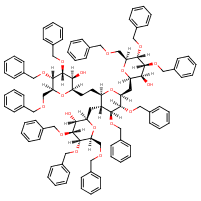Structure InformationCompound Identification

SMILES

O[C@H]1[C@@H](CC[C@H]2O[C@@H](C[C@H]3O[C@@H](COCC4=CC=CC=C4)[C@H](OCC4=CC=CC=C4)[C@H](OCC4=CC=CC=C4)[C@H]3O)[C@@H](OCC3=CC=CC=C3)[C@@H](OCC3=CC=CC=C3)[C@@H]2C[C@H]2O[C@@H](COCC3=CC=CC=C3)[C@H](OCC3=CC=CC=C3)[C@H](OCC3=CC=CC=C3)[C@@H]2O)O[C@@H](COCC2=CC=CC=C2)[C@H](OCC2=CC=CC=C2)[C@@H]1OCC1=CC=CC=C1

InChIKey

InChIKey=XDTINFLUFPJKHA-FZGPXSDISA-N

Formula

C104H114O18

Mass

1652.038

### Export to:

Taxonomic Classification

Taxonomy Tree

Kingdom

Organic compounds

Superclass

Organic oxygen compounds

Class

Organooxygen compounds

Subclass

Carbohydrates and carbohydrate conjugates

Intermediate Tree Nodes

Not available

Direct Parent

Oligosaccharides

Alternative Parents

Molecular Framework

Aromatic heteromonocyclic compounds

Substituents

Oligosaccharide - Glycosyl compound - C-glycosyl compound - Benzylether - Benzenoid - Oxane - Monocyclic benzene moiety - Secondary alcohol - Oxacycle - Organoheterocyclic compound - Ether - Dialkyl ether - Hydrocarbon derivative - Alcohol - Aromatic heteromonocyclic compound

Description

This compound belongs to the class of organic compounds known as oligosaccharides. These are carbohydrates made up of 3 to 10 monosaccharide units linked to each other through glycosidic bonds.

External Descriptors

Not available